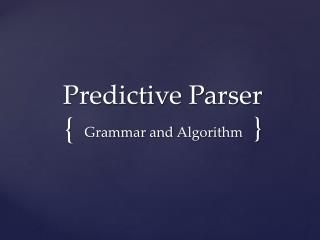DownloadDownload PresentationPredictive Parser

# Predictive Parser

Télécharger la présentation## Predictive Parser

- - - - - - - - - - - - - - - - - - - - - - - - - - - E N D - - - - - - - - - - - - - - - - - - - - - - - - - - -
##### Presentation Transcript

1. Predictive Parser Grammar and Algorithm }

2. Predictive parsers can be constructed for a class of grammars called LL(1). • L->Left L->Leftmost derivation 1->One input symbol at each step • No left recursive or ambiguous grammar can be LL(1) LL(1) Grammar

3. Grammar G is called LL(1) if and only if whenever, If A->α|β are two distinct productions of G, the following conditions hold :- 1. (a) FIRST(α) , FIRST(β) must be disjoint. This is to be able to deterministically guess the production. (b) At most one of the strings αor β can derive ε (Since FIRST(α), FIRST(β) are disjoint. 2. If α -> ε then FIRST(β) and FOLLOW(A) must be disjoint Conditions of LL(1)

4. INPUT :- Grammar G OUTPUT:- Parsing table M For each production A -> α , do the following : • For each terminal ‘a’ in FIRST(A), add A-> α to M[A,α]. • If ε is in FIRST(α) then for each terminal b in FOLLOW(A). A -> α to M[A,b].If b is \$then also add A -> α to M[A,\$]. • If there is no production in M[A,a] , then set M[A,a] to error. Algorithm for construction of parsing table

5. E -> TE’ E -> +TE’|ε T -> FT’ T’ -> *FT’|ε F -> (E)|id Example of LL(1) grammar

6. Generated Parser TableFor String id + id * id

7. A predictive parser can be built by maintaining a stack explicitly. • The table driven parser has an input buffer, stack containing sequence of grammar symbols, parsing table and an output stream. • The input buffer contains the string to be parsed followed by \$. • Initially the stack contains start symbol of the grammar on the top followed by \$. • The parsing table deterministically guesses the correct production to be used. Table driven predictive parsing

8. Flow Chart

9. The current symbol of the input string is maintained by a pointer say ‘ip’. • In every step consider the set {a,α} where ‘a’ is the symbol pointed by the ‘ip’ and ‘α’ is the top of the stack. • If ‘α’ is a Non Terminal ,then see the table cell M{α,a} for the production. 1.If M{α,a} is a valid production then pop the stack , push the production into the stack. 2.If M{α,a} is error or blank then report an error Procedure of predictive parser

10. .If ‘α’ is a terminal then pop it from the stack and also increment the input pointer ‘ip’ to point the next symbol in the input string. • The output will be the set of productions • The following example illustrates the top-down predictive parser using parser table . String: id + id * id Grammar: Mentioned LL(1) Grammar in Previous slides